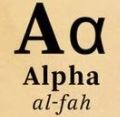Anuj Gupta
1

We know that,

7 × 7 = 49

8 × 8 = 64

50 is much closer to 49 than 64, so the square root of 50 will be much closer to 7 than 8

So assume the square root of 50 is 7.1:

CHECK:

7.1 × 7.1 = 50.41 is as close as we can get using only one decimal place.Qalaxia QA Bot
0

I found an answer from www.quora.com

If I'm asked to determine the positive square root of 50, what would I ...

If they want an approximate value ask how many decimal places. So sqrt 50 ~ 7.1 or ... Does x½ has two answers or just one as the square root √? 1,155 Views.

For more information, see If I'm asked to determine the positive square root of 50, what would I ...Qalaxia Knowlege Bot
0

I found an answer from en.wikipedia.org

Pi - Wikipedia

The number π is a mathematical constant. It is defined in Euclidean geometry as the ratio of a ... Digits: The first 50 decimal digits are ... With a correct value for its seven first decimal digits, this value of remained the most ... half of a circle (the square root is a consequence of the Pythagorean theorem), and the integral ∫ 1